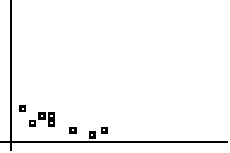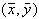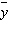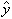Name:    AP Statistics - Chapter 3 MC Study Guide

Multiple Choice
Identify the choice that best completes the statement or answers the question.

1.

In a statistics course, a linear regression equation was computed to predict the final exam score from the score on the first test.  The equation was  y = 10 + .9x  where y is the final exam score and x is the score on the first test.  Carla scored 95 on the first test.  On the final exam, Carla scored 98.  What is the value of her residual?
 a. 98 b. 2.5 c. –2.5 d. 0 e. None of the above

2.

In the scatterplot below, if each x-value were decreased by one unit and the y-values remained the same, then the correlation r woulda. Decrease by 1 unit b. Decease slightly c. Increase slightly d. Stay the same e. Can’t tell without knowing the data values

3.

In regression, the residuals are which of the following?
 a. Those factors unexplained by the data b. The difference between the observed responses and the values predicted by the regression line c. Those data points which were recorded after the formal investigation was completed d. Possible models unexplored by the investigator e. None of the above

4.

Which of the following statements are true?
I.      Correlation and regression require explanatory and response variables.
II.      Scatterplots require that both variables be quantitative.
III.      Every least-square regression line passes through.
 a. I and II only b. I and III only c. II and III only d. I,  II, and III e. None of the above

5.

Suppose the following information was collected, where X = diameter of tree trunk in inches, and Y = tree height in feet.

 X 4 2 8 6 10 6 Y 8 4 18 22 30 8

If the LSRL equation is y = –3.6 + 3.1x, what is your estimate of the average height of all trees having a trunk diameter of 7 inches?
 a. 18.1 b. 19.1 c. 20.1 d. 21.1 e. 22.1

6.

Suppose we fit the least squares regression line to a set of data.  What is true if a plot of the residuals shows a curved pattern?
 a. A straight line is not a good model for the data. b. The correlation must be 0. c. The correlation must be positive. d. Outliers must be present. e. The LSRL might or might not be a good model for the data, depending on the extent of the curve.

7.

Which of the following are resistant?
 a. Least squares regression line b. Correlation coefficient c. Both the least squares line and the correlation coefficient d. Neither the least squares line nor the correlation coefficient e. It depends

8.

A copy machine dealer has data on the number x of copy machines at each of 89 customer locations and the number y of service calls in a month at each location.  Summary calculations give= 8.4, Sx = 2.1,= 14.2, Sy = 3.8, and r = 0.86.  What is the slope of the least squares regression line of number of service calls on number of copiers?
 a. 0.86 b. 1.56 c. 0.48 d. None of these e. Can’t tell from the information given

9.

There is a linear relationship between the number of chirps made by the striped ground cricket and the air temperature.  A least squares fit of some data collected by a biologist gives the model= 25.2 + 3.3x,   9 < x < 25,   where x is the number of chirps per minute andis the estimated temperature in degrees Fahrenheit.  What is the estimated increase in temperature that corresponds to an increase in 5 chirps per minute?
 a. 3.3°F b. 16.5°F c. 25.2°F d. 28.5°F e. 41.7°F

10.

A set of data relates the amount of annual salary raise and the performance rating.  The least squares regression equation is= 1,400 + 2,000x   where y is the estimated raise and x is the performance rating.  Which of the following statements is not correct?
 a. For each increase of one point in performance rating, the raise will increase on average by \$2,000. b. This equation produces predicted raises with an average error of 0. c. A rating of 0 will yield a predicted raise of \$1,400. d. The correlation for the data is positive. e. All of the above are true.